# R abs Function (6 Example Codes) | How to Calculate an Absolute Value

Basic R Syntax:

`abs(x)`

The abs R function computes the absolute value of a numeric data object. The basic syntax for abs in R is illustrated above.

In the following article, I’ll show you six examples for the application of abs in the R programming language.

Let’s get started!

## Example 1: Apply abs Function to Vector

In the first example, I’m going to show you how to get the absolut values of a numeric vector. Let’s first create an example vector:

```x <- c(- 5, 9, 3, - 1, 2)                 # Create example vector
# -5  9  3 -1  2```

Our vector contains five numbers, two of them are negative. To this vector, we can now apply the abs R function:

```x_abs <- abs(x)                           # Apply abs function in R
x_abs                                     # Print output to RStudio console
# 5 9 3 1 2```

Looks good, all values are positive.

By the way, I have recently published a video on my YouTube channel, in which I’m explaining the R code of this example in more detail:

Next, I’m going to show you how to apply abs to more complex data structures.

## Example 2: Absolute Values of Matrix in R

In the second example, I’m going to use the abs command to convert a numeric matrix to only positive values. Let’s create an example matrix:

```mat <- matrix(- 9:5, nrow = 3, ncol = 5)  # Create example matrix
mat                                       # Print output to RStudio console```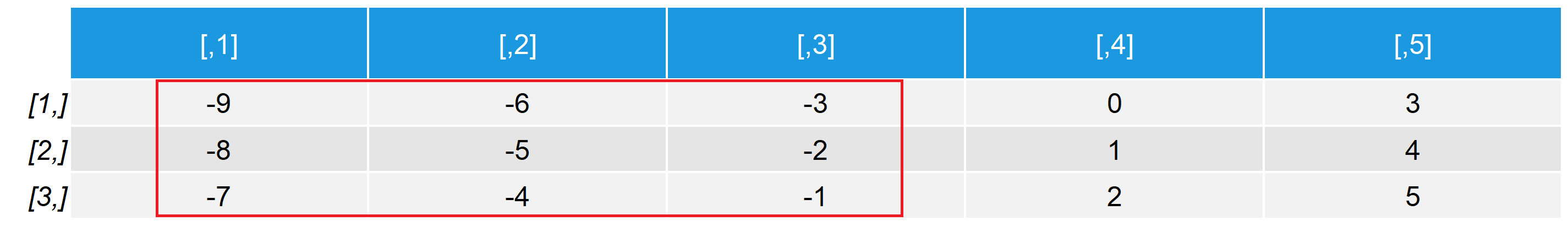Table 1: Example Matrix with Negative Values in Some Data Cells.

Our matrix consists of three rows and five columns. Some of the numbers are negative.

With the abs command, we can replace all negative numbers with their absolute value:

```mat_abs <- abs(mat)                       # Apply abs function to matrix
mat_abs                                   # Print output to RStudio console```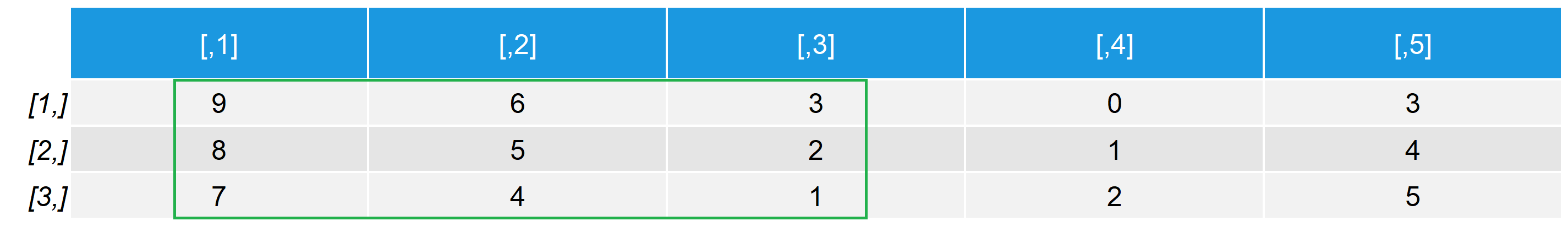Table 2: Matrix with Absolute Values.

As you can see, the updated matrix contains only positive numbers.

## Example 3: Apply abs Function to data.frame

We can do the same conversion as in Example 2 with data.frames. Let’s first convert our previously created matrix to the data.frame class:

```df <- as.data.frame(mat)                  # Convert matrix to data.frame
df                                        # Print output to RStudio console```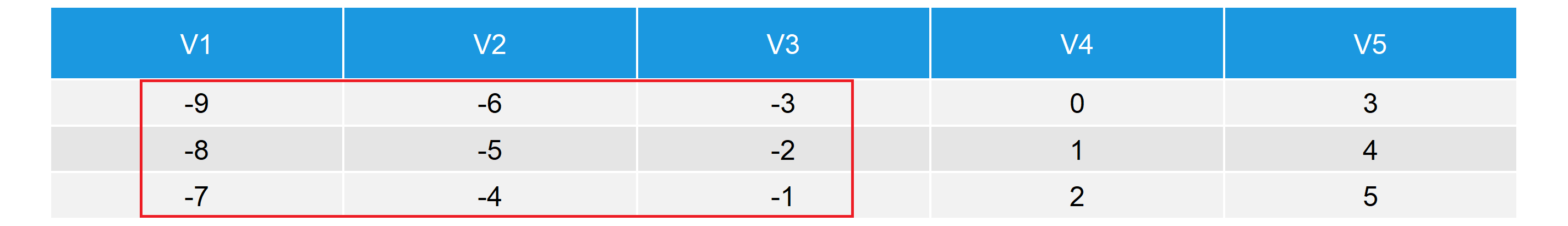Table 3: Example Data Frame with Negative Values in Some Data Cells.

Looks similar as before, but this time our data has column names (i.e. V1-V5).

We can apply the abs function in the same manner as we did before:

```df_abs <- abs(df)                         # Apply abs function to matrix
df_abs                                    # Print output to RStudio console```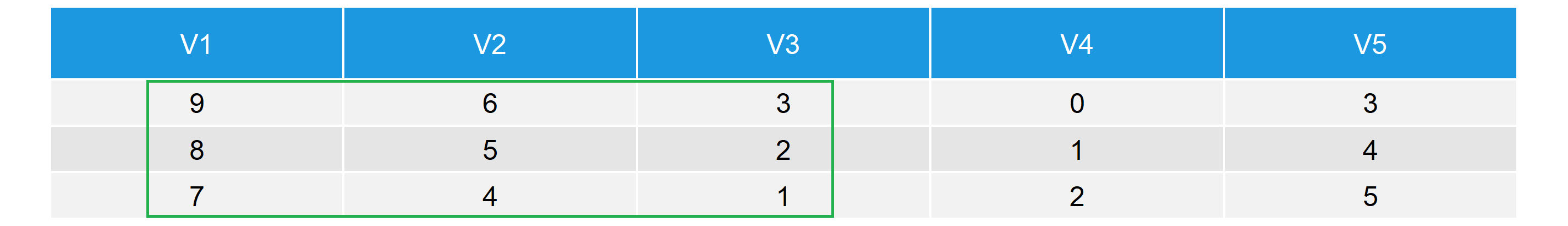Table 4: Data Frame with Absolute Values.

Again, all values are positive.

So what if we want to convert only some values of our data object? That’s exactly what I’m going to show you next.

## Example 4: Calculate Absolute Values of a Column

For this example, I’m using the data.frame of example 3 again. Let’s first create a duplicate of this data…

`df_col_abs <- df                          # Duplicate example data.frame`

…and then let’s calculate the absolute values of column V2:

```df_col_abs\$V2 <- abs(df_col_abs\$V2)       # Absolute value only of one column
df_col_abs                                # Print output to RStudio console```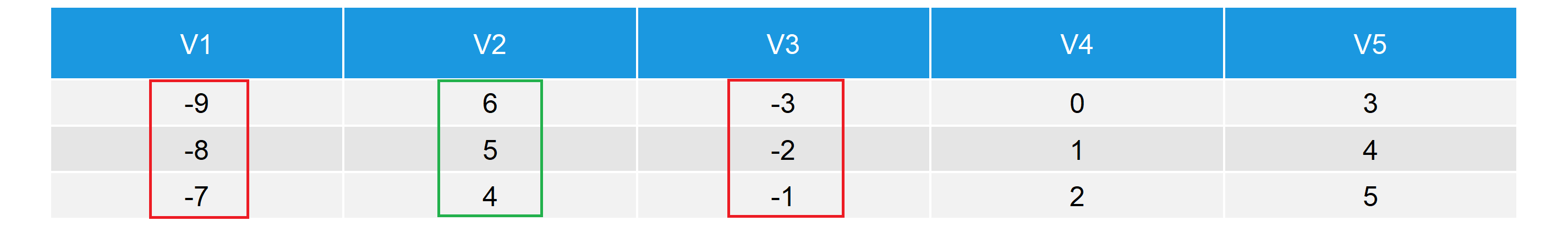Table 5: Data Frame with Absolute Values in One Column.

As you have seen, we can apply the abs function to only one column by using the \$-sign.

Let’s move on with some calculations…

## Example 5: Calculate the Absolute Difference of Two Values

When calculating differences, you might want to know just the absolute difference (not the real difference with a negative value). For this purpose, you can also use the abs function. Let’s first create a data object containing the regular difference of two values:

```diff <- 5 - 20                            # Difference of two values
diff                                      # Print output to RStudio console
# -15```

The data object diff contains the value -15, which is the difference of 5 – 20. If we want to know the absolute difference, we can simply apply the abs function as before:

```diff_abs <- abs(diff)                     # Calculate absolute difference
diff_abs                                  # Print output to RStudio console
# 15```

In this example, we calculated the absolute difference. However, of cause you can calculate the absolute value not only for differences, but also for a correlation, a mean or any other numeric value you can think of.

But there is one restriction…

## Example 6: Error in Math.factor(x) : ‘abs’ not meaningful for factors

The application of abs is in general relatively simple. However, a typical error message is the following:

Error in Math.factor(x) : ‘abs’ not meaningful for factors

So why did this error pop up? Usually, because we tried to apply abs to a factor variable. Let’s do an example…

First, I’m converting the numeric vector of Example 1 to a factor:

```x_fac <- factor(x)                        # Convert example vector to factor
x_fac                                     # Print output to RStudio console
#  -5 9  3  -1 2
# Levels: -5 -1 2 3 9```

If we now apply the abs R command, we get the following error:

```abs(x_fac)                                # Apply abs function to factor
# Error in Math.factor(x_fac) : 'abs' not meaningful for factors```

So if this happens to you, simply convert your factor variable to numeric, before you apply the abs function:

```x_num <- as.numeric(as.character(x_fac))  # Convert factor to numeric
x_num_abs <- abs(x_num)                   # Apply abs command
x_num_abs                                 # Print output to RStudio console
# 5 9 3 1 2```

Works like a charm!

## Video Tutorial: Absolute Value Equations

In this R tutorial, we were speaking much about absolute values. However, we did not learn much about the mathematical concept of absolute values. If you want to learn more about the mathematics of absolute values, I can recommend the following YouTube video of the Khan Academy:

Subscribe to the Statistics Globe Newsletter

•MCLEE
June 28, 2020 11:57 pm

•June 29, 2020 7:25 am